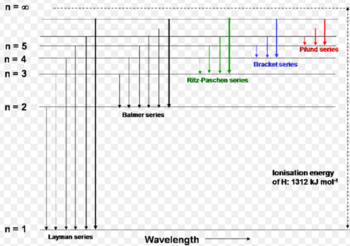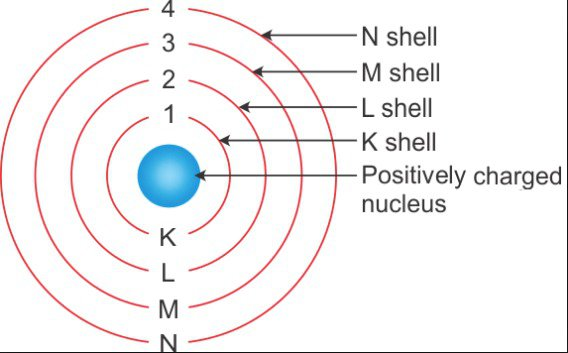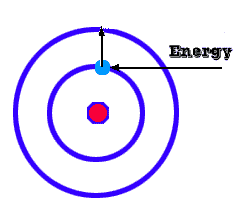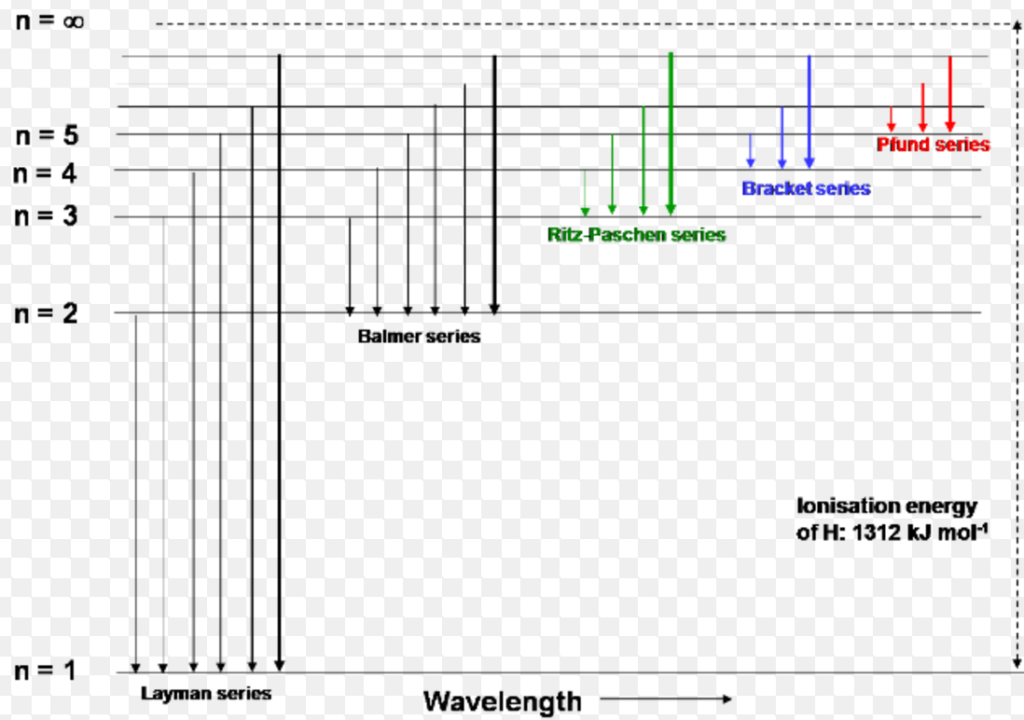Bohr's Atomic TheoryIn 1913, Danish scientist Neils Bohr proposed a model of the atom which was based upon Planck's quantum theory of radiation.

The basic postulates of Bohr's theory are:

1. An atom consists of a small heavy positively charged nucleus in the center surrounded by electrons. The electrons in an atom revolve around the nucleus only in certain selected circular paths, called orbits. These orbits are associated with definite energies and are called shells or energy levels. These are numbered as 1, 2, 3, 4..... etc. Orbits or designated as K, L, M, N ... etc shells.

2. As long as the electron remains in a particular orbit, it does not lose or gain energy. This means that the energy of the electron in a particular energy level remains constant. Therefore, these orbits are also called stationary states. The term stationary does not mean that electron is stationary but it means that the energy of the electron does not change with time. This accounts for the stability of an atom.3. Only those orbits are permitted in which the angular momentum of the electron is a whole number multiple of h/2(pi) ( where h is Planck's constant). An electron, like any other moving body, moving in a circular orbit has an angular momentum equal to mvr, thus, according to Bohr,
mvr=nh/2(pi)                       where m= mass of the electron.
v = velocity.
r = radius of the orbit.
n = 1, 2, 3, 4 .....
This postulate, therefore, introduces the concept of quantization of angular momentum.

When energy is supplied to an atom, its electrons absorb energy and jump to higher energy levels. As soon as the supplied source of energy is cut, the electron jumps back to the original level. A transition from one stationary state to another is accompanied by emission or absorption of energy equal to the difference in energy of the two states. If e is energy in state 1 and E is the energy in state 2, then change of energy E-e in electron jump is given by
E-e=hv.
v=(E-e)/h.
where 'v' is the frequency of the emitted radiations and 'h' is Planck's constant.Interpretation of Hydrogen Spectrum

In hydrogen atom, there is only one electron that is present in the first orbit in the ground state. When energy is supplied, such as bypassing electric discharge, this electron may be excited to some higher energy level. Since in a sample of hydrogen there is a large number of atoms, the electrons in different atoms absorb different amounts of energies and are excited to different higher energy levels. For example, the electrons in some atoms may jump to third energy level while in others it may be raised to fourth energy level and so on. Now, from excited states, the electron may return to the ground state in one or more jumps.

These different downward jumps are associated with different amounts of energies and hence result in the emission of radiations of different wavelengths which appear as different lines in the hydrogen spectrum. The formation of a line spectrum can be interpreted by the fact that the excited electrons in higher energy state are unstable and hence drop back to lower energy state emitting energy in the form of line spectrum. The line spectrum of hydrogen in the visible region is named as Balmer series.

Later studies have shown four other spectral series in the UV and IR regions of the hydrogen spectrum. These are named as spectral series.Limitations of Bohr's Atomic Model

• Bohr's atomic model was quite successful in explaining the stability of atom and line spectrum of hydrogen atom. However, several shortcomings are observed in this model. Some of the important are
• It fails to explain the spectra of atoms containing more than one electron called multielectron system.
• According to Bohr, the circular orbits of the electrons are planar. However, this is not true and the electrons move around the nucleus is three-dimensional space.
• It fails to explain the cause of chemical combination and shapes of the molecules.
• The main objection to Bohr's theory came from the new principles namely the dual nature of electrons and uncertainty principles. This idea of wave character of electron in addition to its particle character over-ruled the Bohr's idea of well defined circular orbits.
• This theory does not explain splitting of spectral lines into a group of finer lines under the influence of magnetic field (Zeeman effect) and electric field ( Stark effect ).